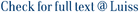In reconstructing the common evolutionary history of hosts and parasites, the current method of choice is the phylogenetic tree reconciliation. In this model, we are given a host tree H, a parasite tree P, and a function σ mapping the leaves of P to the leaves of H and the goal is to find, under some biologically motivated constraints, a reconciliation, that is a function from the vertices of P to the vertices of H that respects σ and allows the identification of biological events such as co-speciation, duplication and host switch. The maximum co-divergence problem consists in finding the maximum number of co-speciations in a reconciliation. This problem is NP-hard for arbitrary phylogenetic trees and no approximation algorithm is known. In this paper we consider the influence of tree topology on the maximum co-divergence problem. In particular we focus on a particular tree structure, namely caterpillar, and show that—in this case—the heuristics that are mostly used in the literature provide solutions that can be arbitrarily far from the optimal value. Then, we prove that finding the max co-divergence is equivalent to compute the maximum length of a subsequence with certain properties of a given permutation. This equivalence leads to two consequences: (1) it shows that we can compute efficiently in polynomial time the optimal time-feasible reconciliation and (2) it can be used to understand how much the tree topology influences the value of the maximum number of co-speciations.

Co-divergence and tree topology / Calamoneri, T.; Monti, A.; Sinaimeri, Blerina. - In: JOURNAL OF MATHEMATICAL BIOLOGY. - ISSN 0303-6812. - 79:3(2019), pp. 1149-1167. [10.1007/s00285-019-01385-w]

### Co-divergence and tree topology

#### Abstract

In reconstructing the common evolutionary history of hosts and parasites, the current method of choice is the phylogenetic tree reconciliation. In this model, we are given a host tree H, a parasite tree P, and a function σ mapping the leaves of P to the leaves of H and the goal is to find, under some biologically motivated constraints, a reconciliation, that is a function from the vertices of P to the vertices of H that respects σ and allows the identification of biological events such as co-speciation, duplication and host switch. The maximum co-divergence problem consists in finding the maximum number of co-speciations in a reconciliation. This problem is NP-hard for arbitrary phylogenetic trees and no approximation algorithm is known. In this paper we consider the influence of tree topology on the maximum co-divergence problem. In particular we focus on a particular tree structure, namely caterpillar, and show that—in this case—the heuristics that are mostly used in the literature provide solutions that can be arbitrarily far from the optimal value. Then, we prove that finding the max co-divergence is equivalent to compute the maximum length of a subsequence with certain properties of a given permutation. This equivalence leads to two consequences: (1) it shows that we can compute efficiently in polynomial time the optimal time-feasible reconciliation and (2) it can be used to understand how much the tree topology influences the value of the maximum number of co-speciations.
##### Scheda breve Scheda completa Scheda completa (DC)2019
caterpillars; co-phylogeny; reconciliations
Co-divergence and tree topology / Calamoneri, T.; Monti, A.; Sinaimeri, Blerina. - In: JOURNAL OF MATHEMATICAL BIOLOGY. - ISSN 0303-6812. - 79:3(2019), pp. 1149-1167. [10.1007/s00285-019-01385-w]
File in questo prodotto:
File
Calamoneri_Co-divergence_2019.pdf

Solo gestori archivio

Tipologia: Documento in Pre-print
Licenza: DRM (Digital rights management) non definiti
Dimensione 549.84 kB
Utilizza questo identificativo per citare o creare un link a questo documento: `https://hdl.handle.net/11385/202521`
•1
•1